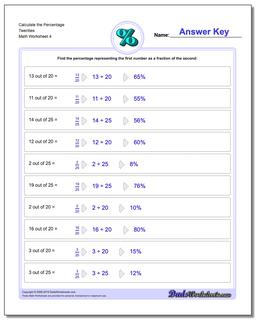# Math Worksheets: Percentages: Percentages: Calculate the Percentage Twenties (Fourth Worksheet)## Calculate the Percentage Twenties (Fourth Worksheet)

PropertyValue
DescriptionCalculate the Percentage Twenties: Percent worksheets where students are given two numbers and must determine what percentage one number is of the other number. (Fourth Worksheet)
Resource TypeWorksheet